Question

# I need help with this  Bode Plots assignment (5 points) Follow the steps (a-c) described below for the following transf...

I need help with this  Bode Plots assignment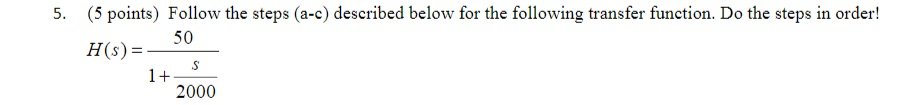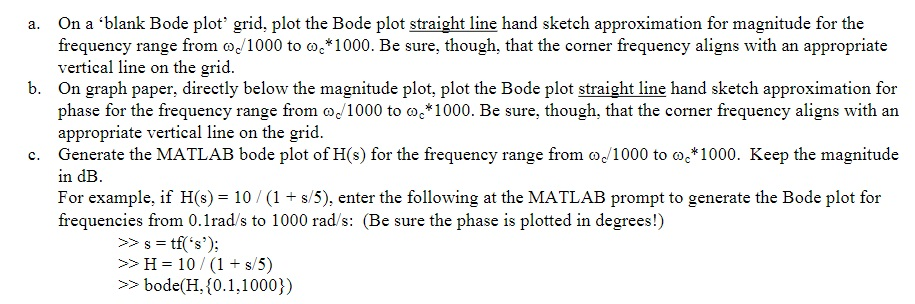(5 points) Follow the steps (a-c) described below for the following transfer function. Do the steps in order! 5. H50 H(s) 2000
a. On a 'blank Bode plot' grid, plot the Bode plot straight line hand sketch approximation for magnitude for the frequency range from 1000 to ω.*1000. Be sure, though, that the corner frequency aligns with an appropriate vertical line on the grid. b. On graph paper, directly below the magnitude plot, plot the Bode plot straight line hand sketch approximation for phase for the frequency range from co1000 to oe 1000. Be sure, though, that the cormer frequency aligns with an appropriate vertical line on the grid c. Generate the MATLAB bode plot of H(s) for the frequency range from o/1000 to o 1000. Keep the magnitude in dB For example, if H(s) 10 (1 + s/5), enter the following at the MATLAB prompt to generate the Bode plot for frequencies from 0. lrad/s to 1000 rad/s: (Be sure the phase is plotted in degrees!) tf(s'); H 10 (1 +s 5) bode(H, 0.1,1000))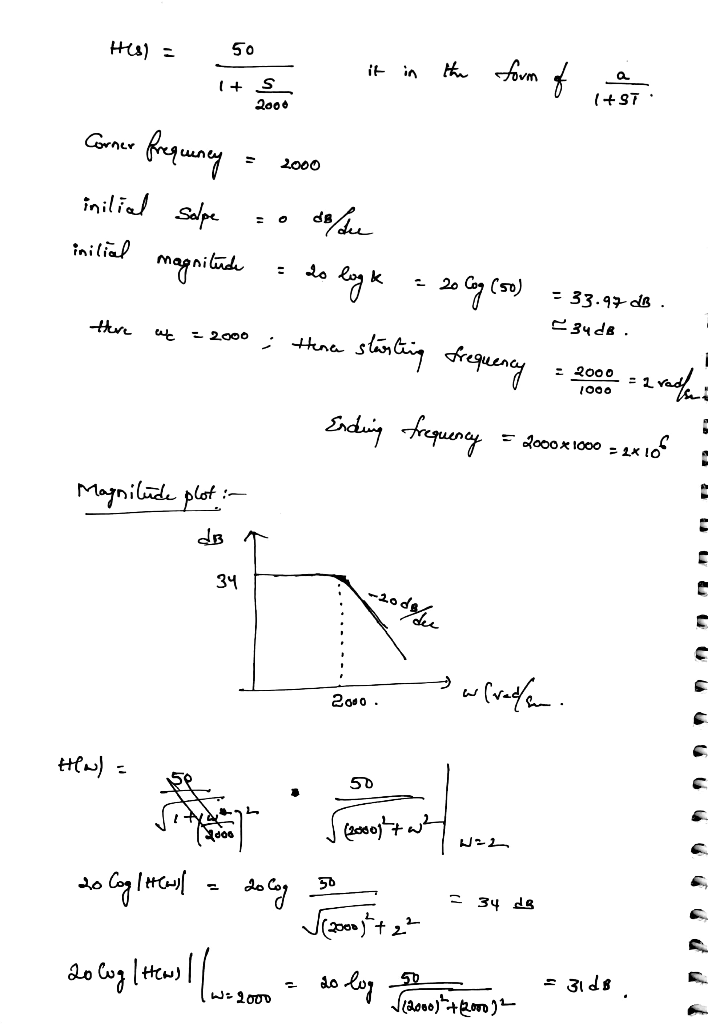matlab code:

clc;clear all;close all;
sys=tf(,[1/2000 1])
bode(sys,{2,2*10^6})

output:

sys =

50
------------
0.0005 s + 1

Continuous-time transfer function.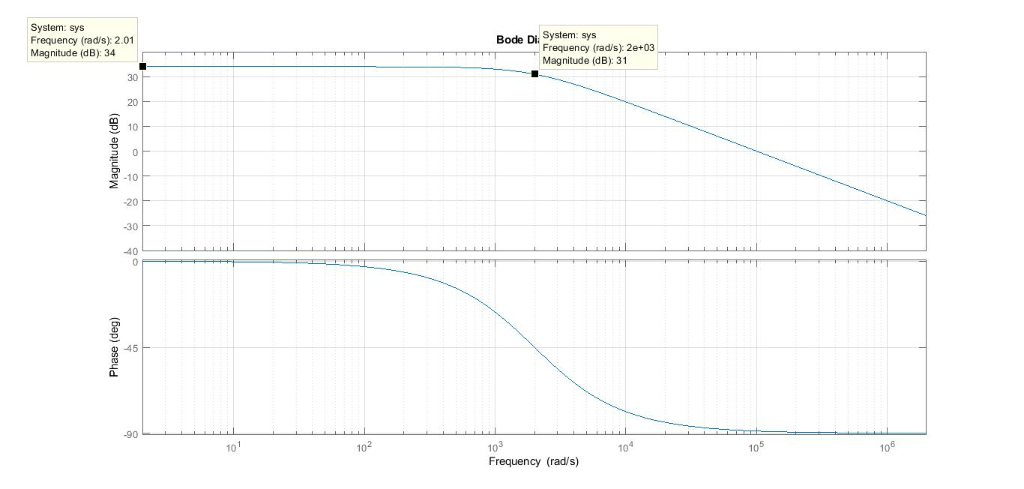#### Earn Coins

Coins can be redeemed for fabulous gifts.

Similar Homework Help Questions
• ### I need help with this  Bode Plots assignment 2. (5 points) Follow the steps (a-c) described below for the following tra...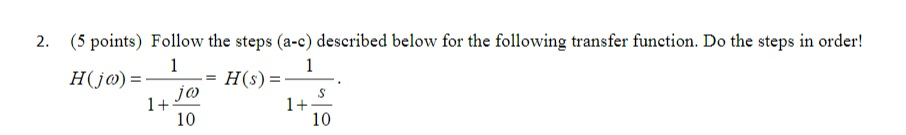I need help with this  Bode Plots assignment 2. (5 points) Follow the steps (a-c) described below for the following transfer function. Do the steps in order! 1+ 10 1+ 10 a. On a 'blank Bode plot' grid, plot the Bode plot straight line hand sketch approximation for magnitude for the frequency range from 1000 to ω.*1000. Be sure, though, that the corner frequency aligns with an appropriate vertical line on the grid. b. On graph paper, directly below the...

• ### Sketch the straight-line approximation Bode plot diagrams (magnitude and phase) 110s for H[s] You might want...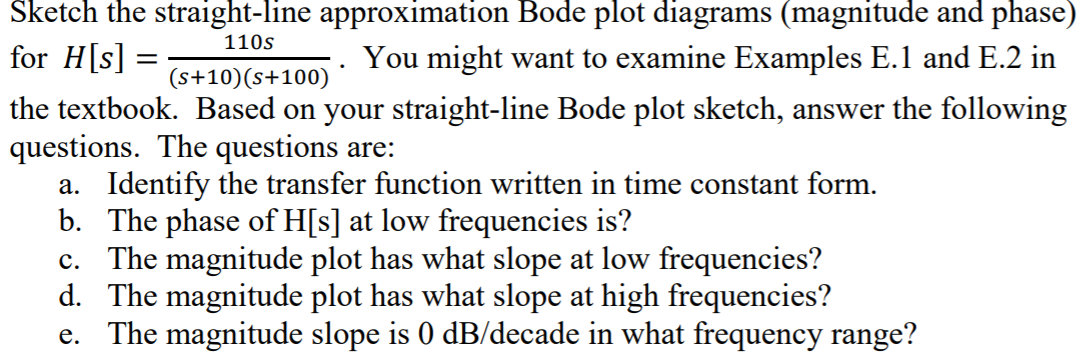Sketch the straight-line approximation Bode plot diagrams (magnitude and phase) 110s for H[s] You might want to examine Examples E.1 and E.2 in (s+10)(s+100)´ the textbook. Based on your straight-line Bode plot sketch, answer the following questions. The questions are: a. Identify the transfer function written in time constant form. b. The phase of H[s] at low frequencies is? c. The magnitude plot has what slope at low frequencies? d. The magnitude plot has what slope at high frequencies? e....

• ### i) Draw the Bode plots (hand sketch, magnitude and phase!) for the following transfer function. Plot...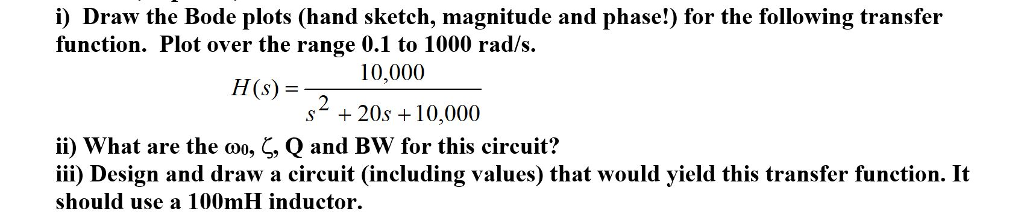i) Draw the Bode plots (hand sketch, magnitude and phase!) for the following transfer function. Plot over the range 0.1 to 1000 rad/s HS 10,000 (s) = s* + 20s 10,000 ii) what are the Q and Bw for this circuit? iii) Design and draw a circuit (including values) that would yield this transfer function. It should use a 100mH inductor , , Qano

• ### a) Please show all your steps. For the magnitude and phase angle plots, approximate bode diagrams...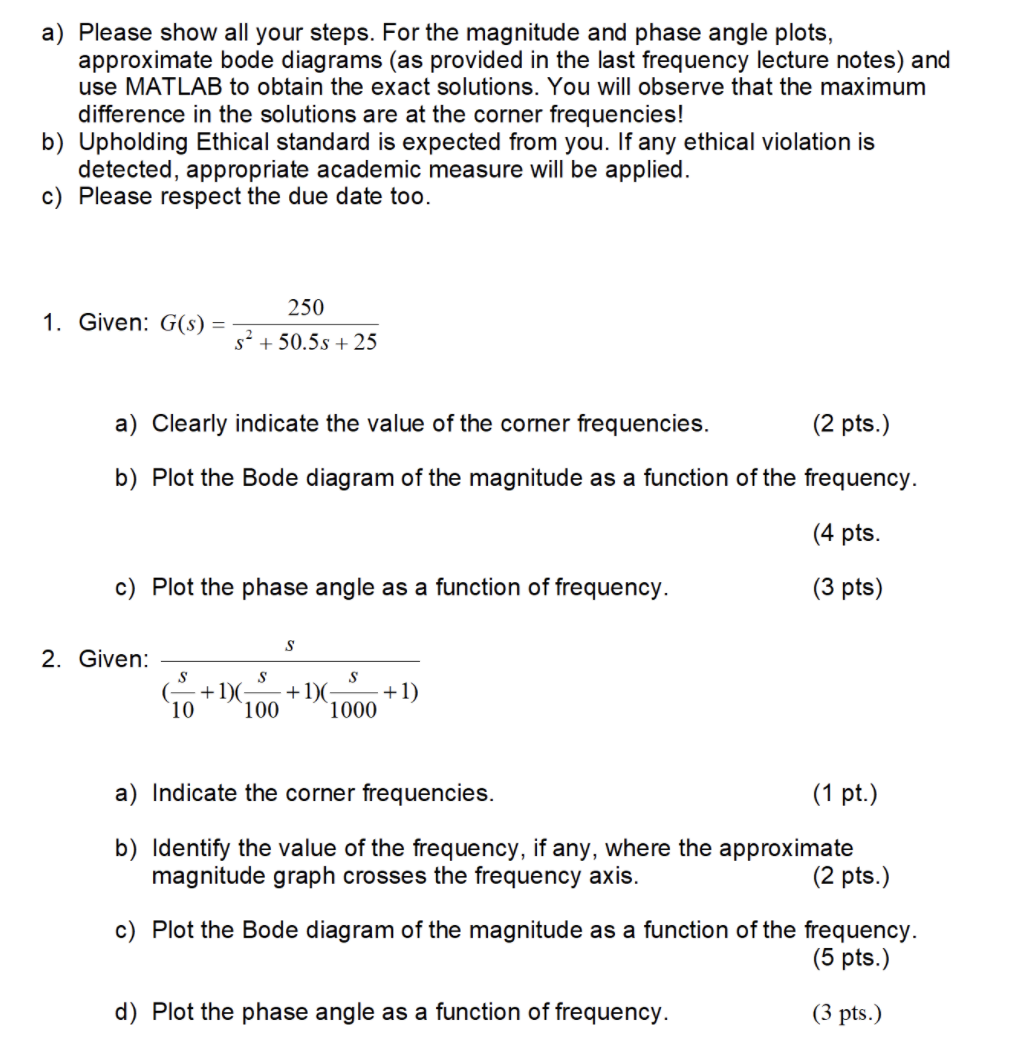a) Please show all your steps. For the magnitude and phase angle plots, approximate bode diagrams (as provided in the last frequency lecture notes) and use MATLAB to obtain the exact solutions. You will observe that the maximum difference in the solutions are at the corner frequencies! b) Upholding Ethical standard is expected from you. If any ethical violation is detected, appropriate academic measure will be applied. c) Please respect the due date too. 250 1. Given: G(S) = 52...

• ### 2. Given the following circuit RL Do where C = inF, L = 1 mH and R1 = į00kf2. (a) Derive the expression for the tra...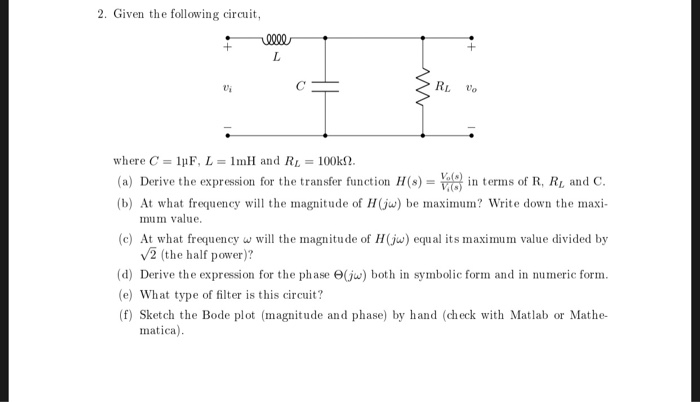2. Given the following circuit RL Do where C = inF, L = 1 mH and R1 = į00kf2. (a) Derive the expression for the transfer functionH(s)0 in erms of R, Ri and C. (b) At what frequency will the magnitude of Hju) be maximum? Write down the maxi- mum value (c) At what frequency w will the magnitude of H(jw) equal its maximum value divided by V2 (the half power)? (d) Derive the expression for the phase e(ju) both...

• ### Please answer all parts Problem #5 - (20%) A circuit has the transfer function: H(S) =...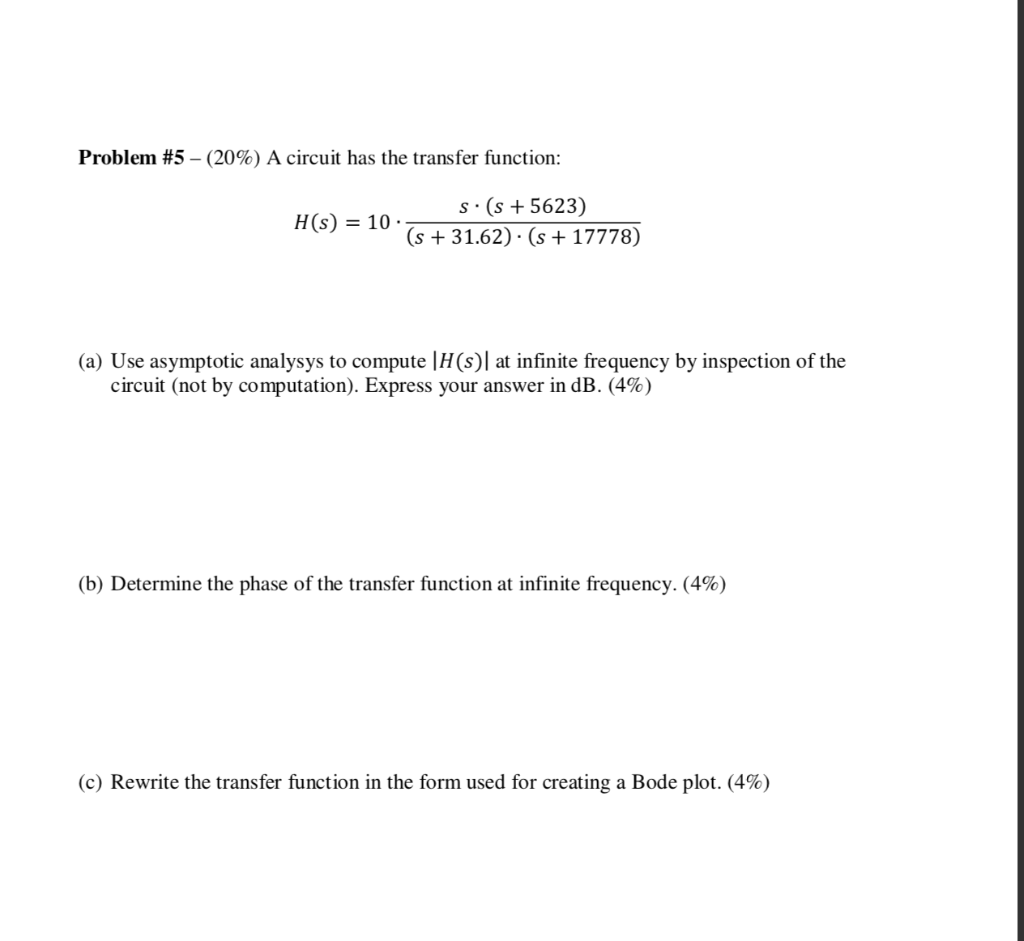Please answer all parts Problem #5 - (20%) A circuit has the transfer function: H(S) = S. (s + 5623 (s + 31.62) · (s + 17778) (a) Use asymptotic analysys to compute (HS) at infinite frequency by inspection of the circuit (not by computation). Express your answer in dB. (4%) (b) Determine the phase of the transfer function at infinite frequency. (4%) (c) Rewrite the transfer function in the form used for creating a Bode plot. (4%) Problem #5...

• ### I need help with the following signals problems for parts A, B, C, and D. Thanks...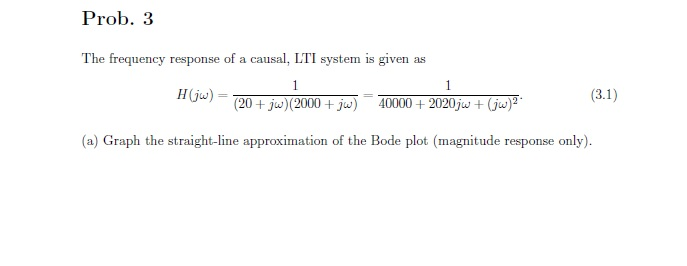I need help with the following signals problems for parts A, B, C, and D. Thanks in advanced. Prob. 3 The frequency response of a causal, LTI system is given as Hj (20 + ju)2000ju0000+ 2020js+) (a) Graph the straight-line approximation of the Bode plot (magnitude response only). 10 1.401080 100 100000 10 10000 ω (rades) Prob. 3 (cont.) (b) Determine the linear, constant-coefficient differential equation (LCCDE) of the system. (c) Draw a block diagram of the system using differentiators...

• ### Problem 1 Consider the following asymptotic Bode plots. Note that the frequencies are in Hz. For...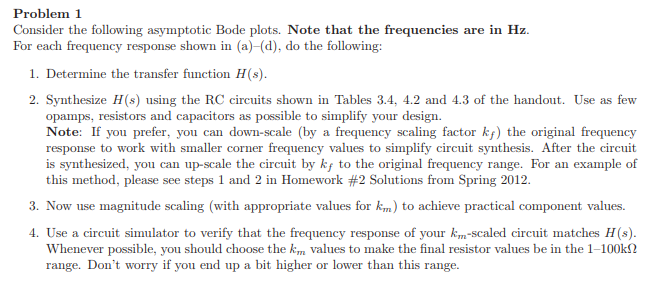Problem 1 Consider the following asymptotic Bode plots. Note that the frequencies are in Hz. For each frequency response shown in (a)-(d), do the following: 1. Determine the transfer function H(s). 2. Synthesize H(s) using the RC circuits shown in Tables 3.4, 4.2 and 4.3 of the handout. Use as few opamps, resistors and capacitors as possible to simplify your design. Note: If you prefer, you can down-scale (by a frequency scaling factor ky) the original frequency response to work...

• ### Clear steps, please. Consider the following LTI system where, Q -5, and w 2000m rad./sec. a)...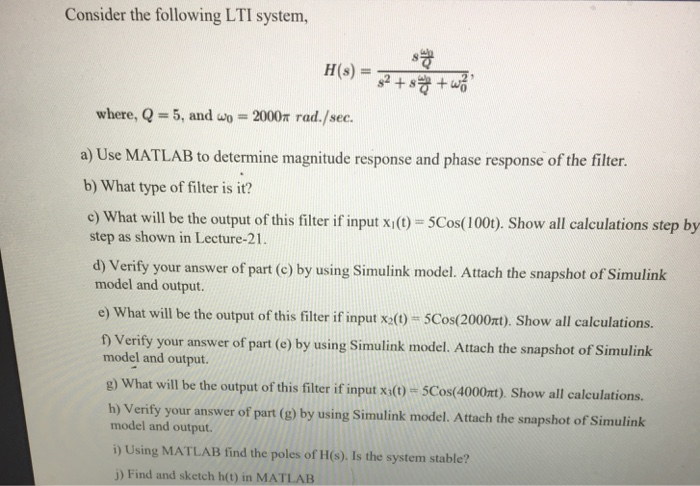Clear steps, please. Consider the following LTI system where, Q -5, and w 2000m rad./sec. a) Use MATLAB to determine magnitude response and phase response of the filter. b) What type of filter is it? c) What will be the output of this filter if input xio- 5Cos(1000). Show all calculations step by step as shown in Lecture-21 . d) Verify your answer of part (e) by using Simulink model. Attach the snapshot of Simulink model and output. e) What...

• ### I need help with number 2 . Please answer in MATLAB thank you! 1. In the lab we analyzed filtering 60 Hz power-line noi...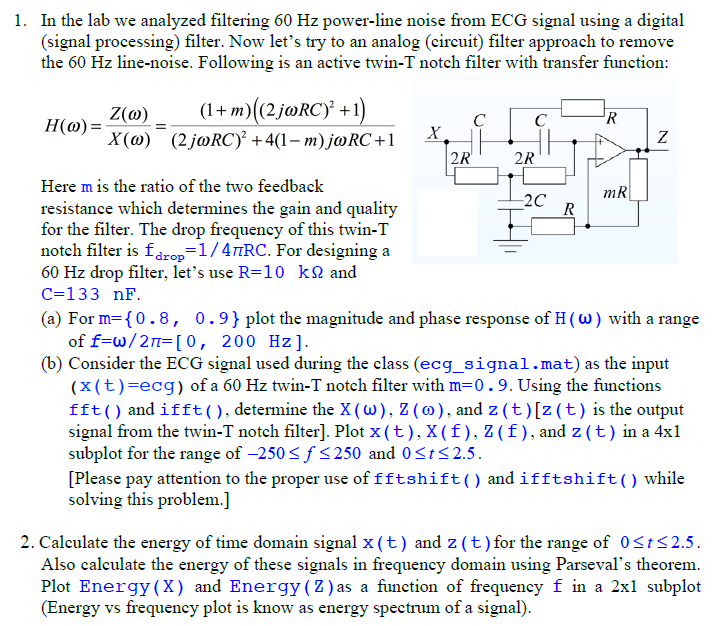I need help with number 2 . Please answer in MATLAB thank you! 1. In the lab we analyzed filtering 60 Hz power-line noise from ECG signal using a digital (signal processing) filter. Now let's try to an analog (circuit) filter approach to remove the 60 Hz line-noise. Following is an active twin-T notch filter with transfer function: (1+m)((2joRC? +1 Z(a) 2R 2R Here m is the ratio of the two feedback 2C R resistance which determines the gain and...